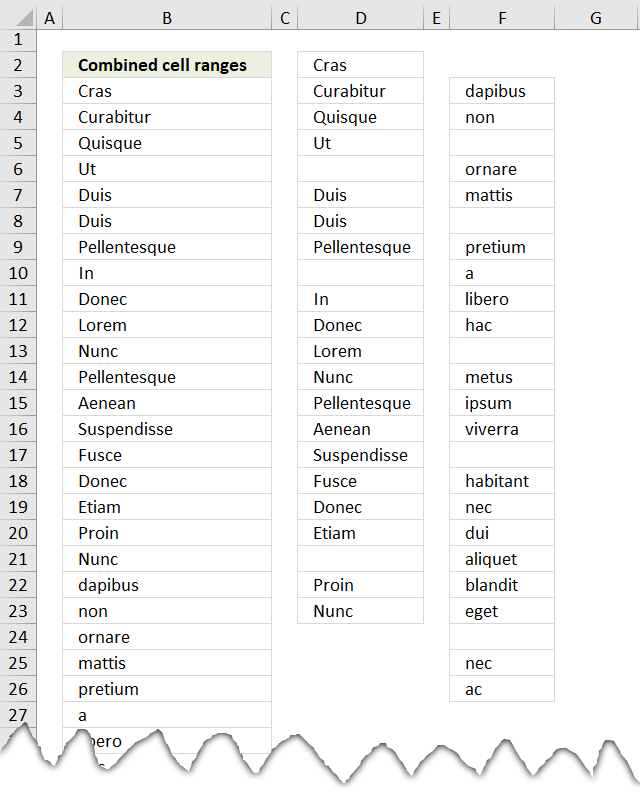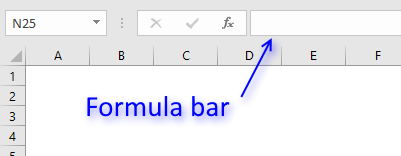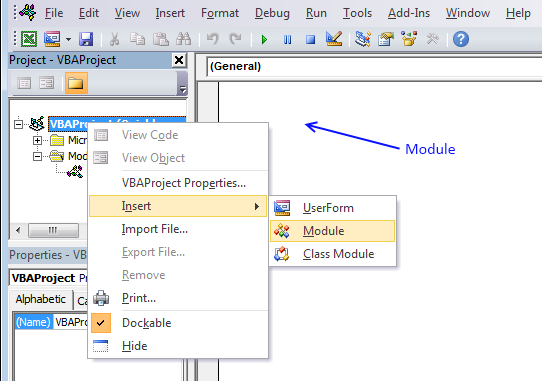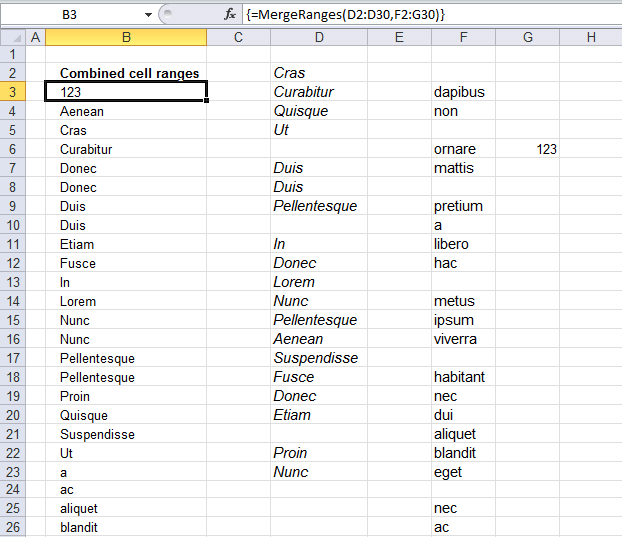Author: Oscar Cronquist Article last updated on May 16, 2019The image above demonstrates a user defined function that merges up to 255 cell ranges and removes blanks. I will also show you how to sort these values.

A user defined function is a custom function in Excel than anyone can build, you simply copy the code below to a module in the Visual Basic Editor and then enter the function name and arguments in a cell.

I have articles that shows you how to combine two and three columns using array formulas. Check out this article that demonstrates how to Merge tables based on a condition.

Array formula in cell range B3:B70:

=MergeRanges(D2:D30, F2:F30, Sheet2!C1:C35)

#### How to create an array formula

1. Select cell range B3:B70.
2. Copy (Ctrl + c) above array formula.
3. Paste (Ctrl + v) array formula to formula bar.4. Press and hold Ctrl + Shift.
5. Press Enter once.
6. Release all keys.

#### VBA

```'Name function and declare arguments
Function MergeRanges(ParamArray arguments() As Variant) As Variant()
'Declare variables and data types
Dim cell As Range, temp() As Variant, argument As Variant
Dim iRows As Integer, i As Integer
'Redimension temp in order to let it grow if needed
ReDim temp(0)
'Iterate through each cell range
For Each argument In arguments
'Iterate through each cell in cell range
For Each cell In argument
'If cell not equal to nothing
If cell &lt;&gt; "" Then
'Save cell value to array variable
temp(UBound(temp)) = cell
ReDim Preserve temp(UBound(temp) + 1)
End If
Next cell
Next argument
'Remove container from array
ReDim Preserve temp(UBound(temp) - 1)
'Count cells occupied by user defined function
For i = UBound(temp) To iRows
ReDim Preserve temp(UBound(temp) + 1)
'Save "" to array
temp(UBound(temp)) = ""
Next i
'Return array
MergeRanges = Application.Transpose(temp)

End Function
```1. Press Alt-F11 to open visual basic editor
2. Click Module on the Insert menu
3. Copy and paste vba code
4. Exit visual basic editorCombine-ranges.xls

Sort text from multiple cell ranges combined (user defined function):

Sort text cells alphabetically from two columns

Table of Contents Sort text from two columns combined (array formula) Sort text from multiple cell ranges combined (user defined […]

### Merge,sort and remove blanks from multiple cell rangesI used the "Sort array" function found here: Using a Visual Basic Macro to Sort Arrays in Microsoft Excel (microsoft) with some small modifications.

```Function SelectionSort(TempArray As Variant)
Dim MaxVal As Variant
Dim MaxIndex As Integer
Dim i As Integer, j As Integer

' Step through the elements in the array starting with the
' last element in the array.
For i = UBound(TempArray) To 0 Step -1

' Set MaxVal to the element in the array and save the
' index of this element as MaxIndex.
MaxVal = TempArray(i)
MaxIndex = i

' Loop through the remaining elements to see if any is
' larger than MaxVal. If it is then set this element
' to be the new MaxVal.
For j = 0 To i
If TempArray(j) > MaxVal Then
MaxVal = TempArray(j)
MaxIndex = j
End If
Next j

' If the index of the largest element is not i, then
' exchange this element with element i.
If MaxIndex < i Then
TempArray(MaxIndex) = TempArray(i)
TempArray(i) = MaxVal
End If
Next i

End Function
```